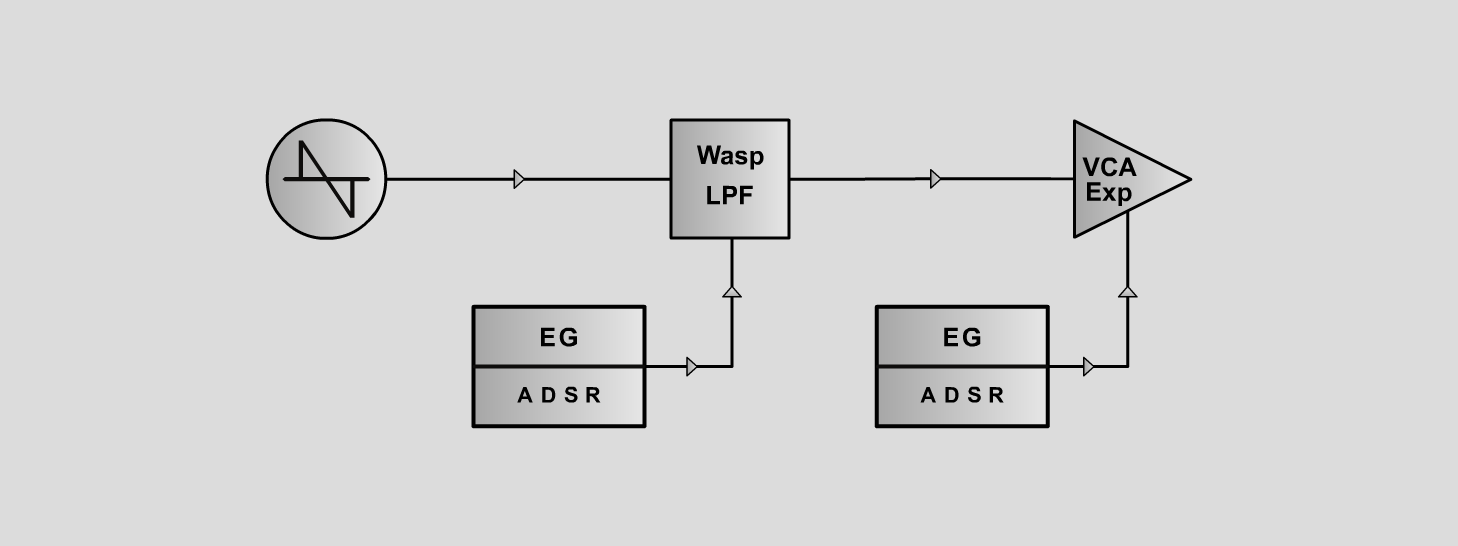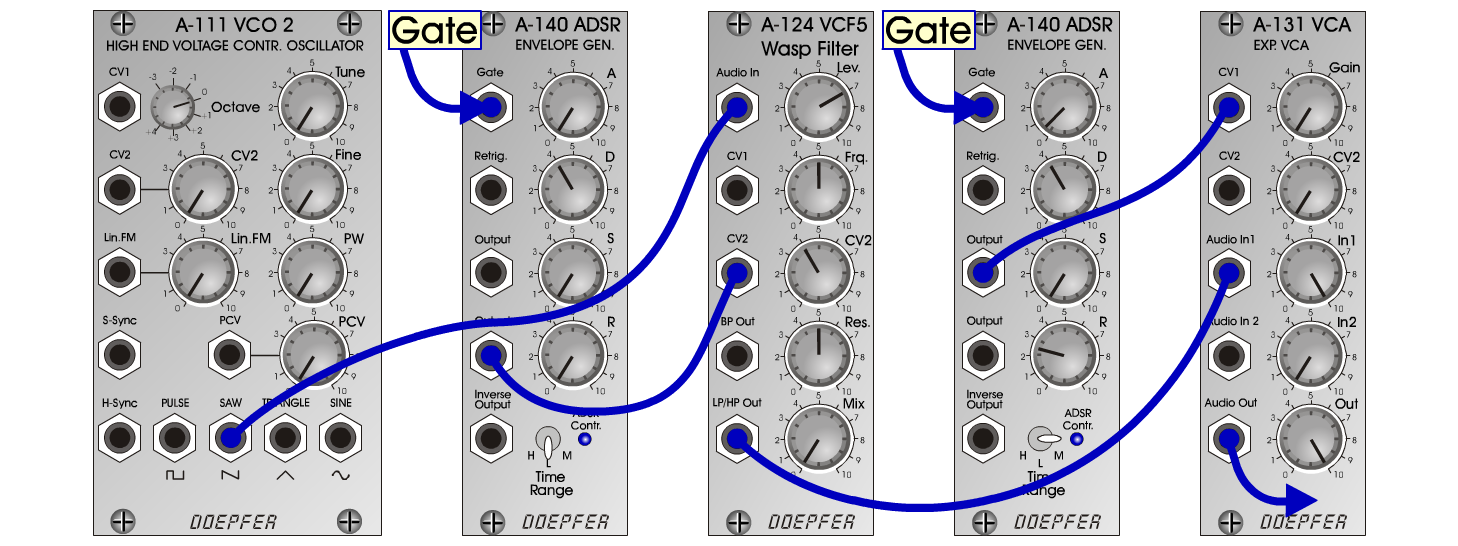WASP-12bS y n t hD o e p f e r A - 100 Connections: Settings: WASP-12b - 1 A-111 (Saw) <=> A-124 (Audio In)   A-140/1 (Output) <=> A-124 (CV2)   A-124 (LP/HP Out) <=> A-131 (Audio In)   A-140/2 (Output) <=> A-131 (CV1) A-124 (Lev = 7, Frq = 5, CV2 = 4, Res = 5, Mix = 0)   A-140/1 (A = 0, D = 4, S = 0, R = 0, Range = L)   A-140/2 (A = 0.5, D = 4, S = 0, R = 2.5, Range = M)   A-131 (Gain = 0, Audio In 1 = 10, Audio Out = 10) WASP-12b - 2 A-124 (Lev = 7, Frq = 4, CV2 = 7, Res = 5, Mix = 0)   A-140/1 (A = 0, D = 8, S = 0, R = 0, Range = L)   A-140/2 (A = 3, D = 5, S = 0, R = 10, Range = M)   A-131 (Gain = 0, Audio In 1 = 10, Audio Out = 10) Josef MuellerSound samples WASP-12b 1 (65 Hz) WASP-12b 1 (131 Hz) WASP-12b 1 (262 Hz) WASP-12b 2 (65 Hz) WASP-12b 2 (131 Hz) WASP-12b 2 (262 Hz)